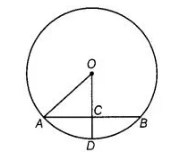# In figure, if OA = 5 cm,`
Question:

In figure, if OA = 5 cm, AB = 8 cm and OD is perpendicular to AB, then CD is equal to(a) $2 \mathrm{~cm}$

(b) $3 \mathrm{~cm}$

(c) $4 \mathrm{~cm}$

(d) $5 \mathrm{~cm}$

Solution:

(a) We know that, the perpendicular from the centre of a circle to a chord bisects the chord.

AC = CB = ½ AB = ½ x 8 = 4 cm

given OA = 5 cm

AO2 = AC2 + OC2

(5)2 = (4)2 + OC2

25 = 16 + OC2

OC2 = 25-16 = 9

OC = 3 cm

[taking positive square root, because length is always positive]

OA = OD [same radius of a circle]

OD = 5 cm

CD = OD – OC = 5 – 3 = 2 cm